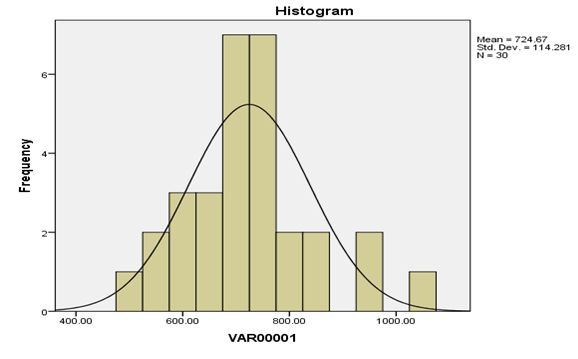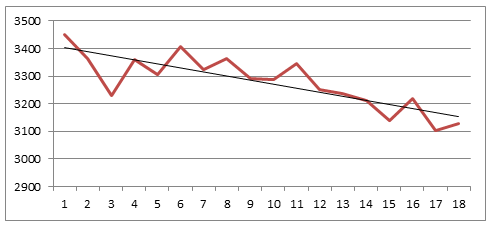# STA101 Statistics for Business Paper Editing Service## Question 1

1. Gender – Categorical and Nominal

2. Education – Categorical and Ordinal

3. Hours of work – Continuous numerical and Interval

4. Number of children – discrete numerical and interval

5. Parents – cateorical and interval### Question 2

A. Mean, Median and Standard deviation

 Mean 724.67 Median 720.00 Mode 730.00 Standard Deviation 114.28 Sample Variance 13,060.23 Kurtosis 0.94 Skewness 0.64

The mode as the data integer value is in the small range of nearly 30 different data types

B. Yes, the measure agree to the central tendency

C. Standard deviation:114.28

D. Sort and Standardise the data

 500 560 570 600 620 620 650 660 670 690 690 700 700 710 720 720 730 730 730 730 740 740 760 800 820 840 850 930 930 1,030

E. Unusual data valuesYes, there are outliers which is clearly noted in the above histogram chart

F. Normal distribution

 Mean 724.67 Standard Deviation 114.28 Kurtosis 0.94 Skewness 0.64 Count 30

The normal distribution possess a oveall skewness of 0 and a kurtosis of 3, From the above table the value of Skewness is 0.64 and kurtosis is 0.94. Therefore the data is normal distribution.

### Question 3

Part a

 Mean Median Mode Quiz 1 72 72 60 Quiz 2 72 72 65 Quiz 3 76 72 72 Quiz 4 76 86.5 90

#### Part b

From the analysis the measure of central tendency agree on Quiz 1, Quiz 2 and Quiz 3. However, the Quiz 4 is not agreeing to central tendency.

#### Part c

Mean uses all the data and information in determining the total output, however a very large data or few data may affect the overall output

Median imparts that the high value or the small value will not affect the values like mean, however it will take more time to compute the value

Mode uses only average values and sometimes there may be more than one value of mode.

#### Part d

 Skewness Kurtosis Quiz 1 1.01 0.50 Quiz 2 0.00 -2.21 Quiz 3 1.57 1.18 Quiz 4 -1.87 3.40

From the above table the skewness of Quiz 3 is 0, therefore the data is symmetric and it is normally distributed, however the values of quiz 1 and quiz 3 is 1.01 and 1.57 respectively, since the value is positive it is rightly skewed (positively skewed) and the skewness of quiz 4 is negatively skewed (skewed left).

#### Part e

From the analysis it is noted that mean and median of Quiz 1 and Quiz 2 possess the same mean and median but the mode is less which shows that the distribution is skewed to the right, however in case of quiz 3 the mean is greater than the median and mode so it is negatively skewed. The quiz 4 shows that the mean is lesser than the median and median is lower than the mode which shows it is skewed to the right.

### Question 4

The overall probability that a given alternator may fail during the 1 hour journey is 0.02, this shows that the probability not failing is 0.98

Probability that both will fail

= 0.02 x 0.02

= 0.0004

Probability that both will not fail

= 0.98 x 0.98

= 0.9604

Probability that one will fail

Probability the first will fail and the second will not fail

= 0.02 x 0.98

= 0.0196

Probability that first will not fail and second will fail

= 0.98 x 0.02

= 0.0196

Therefore that the probability that either one or other will fail

= 0.0196 + 0.0196

= 0.0392

### Question 5

#### Part a

95% confidence interval

= [(mean – 1.96 *(stdev/squareroot (18), mean + 2.11.96098 *(stdev/squareroot (18)]

=[(3278.72 – 1.96 x 97.05 / 4.24), (3278.72 – 1.96 x 97.05 / 4.24)]

= [(3278.72 - 44.84), (3,278.72 + 44.840]

=[3,233.89, 3,323.56]

Therefore it can be stated that the 95% confidence interval is 3,233 and 3,323 steps

#### Part b

Sample size

= (t x standard deviation / error) ^ 2

= (1.95 x 97.05 / 20)^ 2

= 89.5 days

This shows that the data required is nearly 90 days

#### Part c### References

Freedman, David (2010). Statistics. 4th Edition. Cengage Publishing

Sarah Boslaugh (2012). Statistics in a Nutshell. Cengage Publishing

Sincich T. Terry (2012). Statistics. 12th Edition.

Witte S. Robert, (2010). Statistics. 5th Edition. McGraw Hill
Triola (2014). Essentials of Statistics. 5th Edition. McGraw Hill